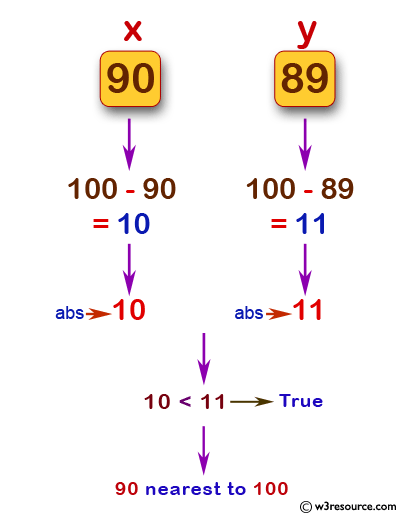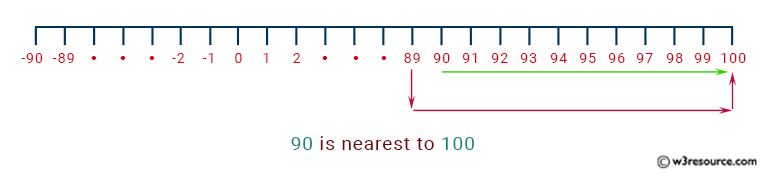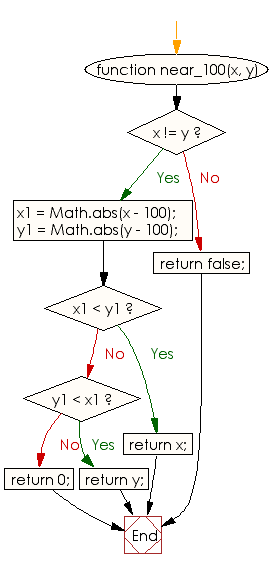# JavaScript: Find a value which is nearest to 100 from two different given integer values

## JavaScript Basic: Exercise-32 with Solution

Write a JavaScript program to find a value which is nearest to 100 from two different given integer values.

Pictorial Presentation - 1:Pictorial Presentation - 2:Sample Solution:

HTML Code:

``````<!DOCTYPE html>
<html>
<meta charset="utf-8">
<meta name="viewport" content="width=device-width">
<title>JavaScript program to find a value which is nearest to 100 from two different given integer values.</title>
<body>

</body>
</html>
```
```

JavaScript Code:

``````function near_100(x, y) {
if (x != y)
{
x1 = Math.abs(x - 100);
y1 = Math.abs(y - 100);

if (x1 < y1)
{
return x;
}
if (y1 < x1)
{
return y;
}
return 0;
}
else
return false;
}

console.log(near_100(90, 89));
console.log(near_100(-90, -89));
console.log(near_100(90, 90));
```
```

Sample Output:

```90
-89
false
```

Flowchart:ES6 Version:

``````function near_100(x, y) {
if (x != y)
{
x1 = Math.abs(x - 100);
y1 = Math.abs(y - 100);

if (x1 < y1)
{
return x;
}
if (y1 < x1)
{
return y;
}
return 0;
}
else
return false;
}

console.log(near_100(90, 89));
console.log(near_100(-90, -89));
console.log(near_100(90, 90));
``````

Live Demo:

See the Pen JavaScript: value which is nearest to 100 - ex-32 by w3resource (@w3resource) on CodePen.

Improve this sample solution and post your code through Disqus

What is the difficulty level of this exercise?

Test your Programming skills with w3resource's quiz.

﻿

## JavaScript: Tips of the Day

Returns the sum of the powers of all the numbers from start to end (both inclusive)

Example:

```const sumPower = (end, power = 2, start = 1) =>
Array(end + 1 - start)
.fill(0)
.map((x, i) => (i + start) ** power)
.reduce((a, b) => a + b, 0);
console.log(sumPower(10)); // 385
console.log(sumPower(10, 3)); // 3025
console.log(sumPower(10, 3, 5)); // 2925
```

Output:

```385
3025
2925
```